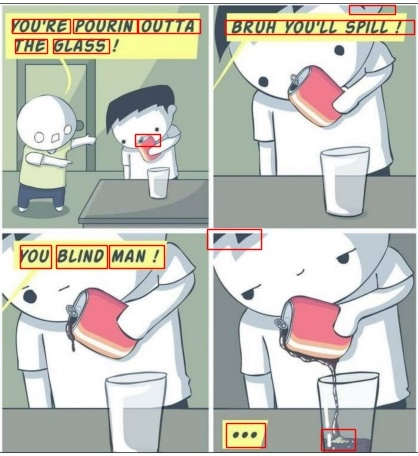# Extracting Text Regions from an image using Geometric properties

The problem of building systems that mimic human behavior is not an easy one to solve. Neural Nets solve those problems in one way but if someone thinks that it’s the right way doesn’t know enough about Artificial Intelligence. When you show a child a picture of a dog, just one picture, the child can locate the dog of any form in any other picture. This is not how neural networks work. We need 1000s to pictures of dogs to build a model that can locate a dog in a picture. And the accuracy of this system is heavily reliant on the quality and size of the dataset, and if you don’t have enough data, you can’t even train a Neural Network system, which basically means you don’t have a solution.

I believe our brain is an advanced pattern matching system. The right way to solve any computer vision problem is to find algorithms that are close to how the brain does it. A pattern matching based solution doesn’t need too much data which is why I love them.

In this post, I will attempt to solve the problem of Text Segmentation using only geometric properties of components in the image to separate text regions from non-text regions and draw bounding boxes around them. The core idea is that Text usually has a lot of common characteristics. You can find the entire project for text detector here https://github.com/muthuspark/text-detector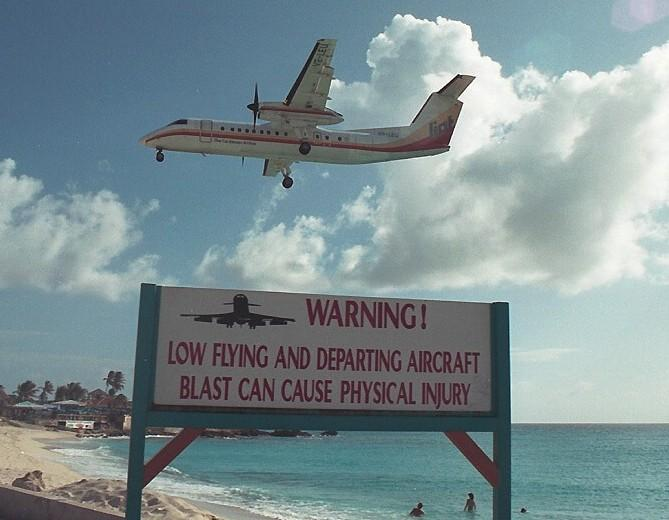I will attempt to run my algorithm over the above sample image to extract text regions from it.

## Algorithm

1. Binarize the image using an Adaptive Thresholding algorithm.
2. Find connected components in the image and Identify likely text regions using Heuristics filter.
3. Draw bounding boxes around the likely text regions and Increase the size of bounding boxes so that they overlap neighboring boxes.
4. Combine the overlapping boxes and remove the boxes which are not overlapping with any other box.
5. You will be left with a few bounding boxes which can be sent to an OCR system like Tesseract.

### Step 1: Binarize the image using an Adaptive Thresholding algorithm.

Load the image and binarize it using an Adaptive Thresholding algorithm. Generally, there are two approaches to binarize a grayscale image: Global threshold and Local threshold. Adaptive thresholding is a form of thresholding that takes into account spatial variations in illumination which is the case in most of our datasets.

```from skimage.filters import threshold_sauvola

thresh_sauvola = threshold_sauvola(img, window_size=window_size)
binary_sauvola = img < thresh_sauvola```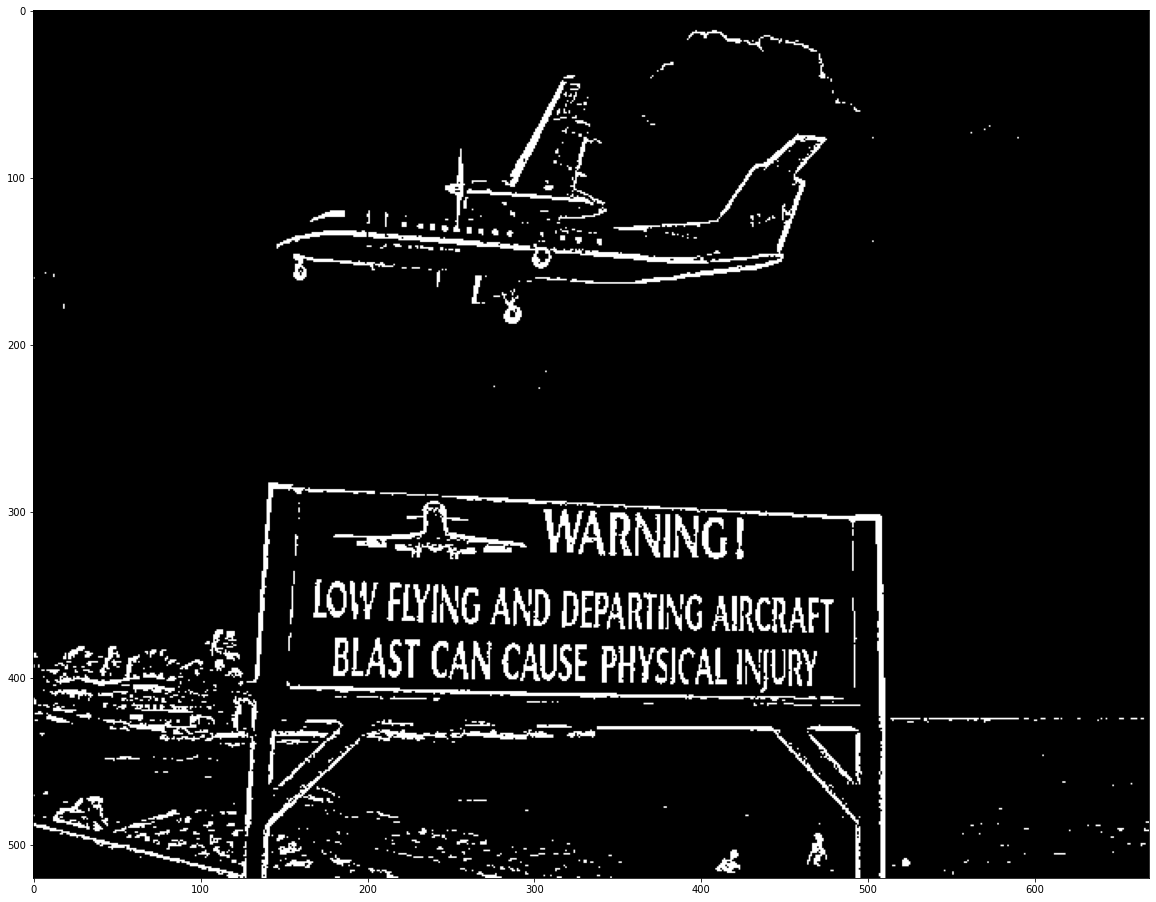### Step 2: Find connected components in the image and Identify likely text regions using Heuristics.

We will find the connected components in the image and filter out the non-text regions using few geometric properties which can help us discriminate between text and non-text regions. Some of the well-known properties mentioned in many research papers are:

1. When components have a low area ( area < 15 ), they are usually noise and are hardly legible.
2. When the density of the component is too low, it can be a diagonal or a noise element (the normal density of text element is usually greater than 20%)
3.  Aspect ratio,  the ratio of the height and the width should not be too low or too high.
4. Eccentricity measures the shortest length of the paths from a given vertex v to reach any other vertex w of a connected graph.
5. Extent, the proportion of pixels in the bounding box that is also in the region. Computed as the Area divided by the area of the bounding box.
6. Solidity, also known as convexity. The proportion of the pixels in the convex hull that are also in the object. Computed as Area/ConvexArea.
7. Stroke Width uniformity – Text characters usually have a uniform stroke width across. This is an important geometric property which is a major contributor to our algorithm.
```aspect_ratio = width/height

should_clean = region.area < 15
should_clean = should_clean or aspect_ratio < 0.06  or aspect_ratio > 3
should_clean = should_clean or region.eccentricity > 0.995
should_clean = should_clean or region.solidity < 0.3
should_clean = should_clean or region.extent < 0.2 or region.extent > 0.9

# stroke width
strokeWidthValues = distance_transform_edt(region.image)
strokeWidthMetric = np.std(strokeWidthValues)/np.mean(strokeWidthValues)
should_clean = should_clean or strokeWidthMetric < 0.4

if not should_clean:
# record the bounding boxes which are highly likely text.
bounding_boxes.append([minr, minc, maxr, maxc])```

#### All connected components#### Connected components after geometric properties based filtering.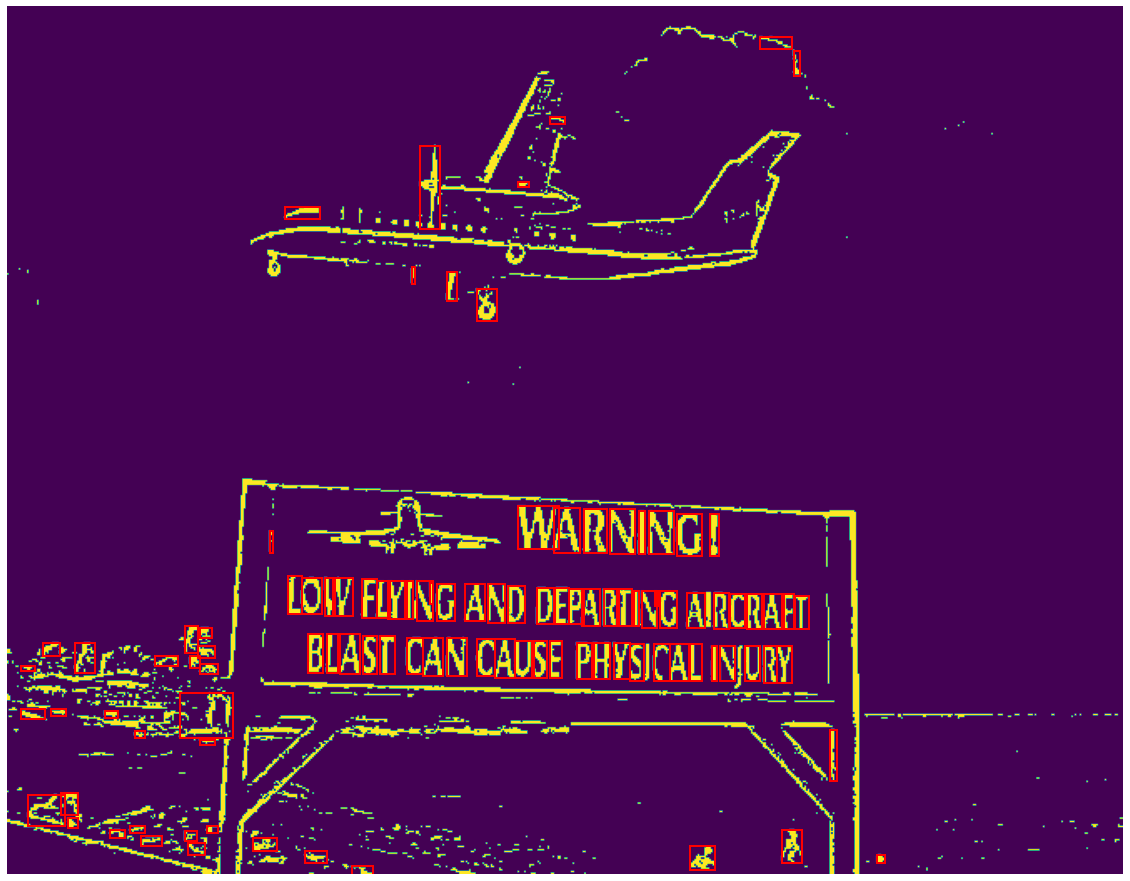### Step 3: Draw bounding boxes around the likely text regions and Increase their size.

Once we have identified the bounding boxes we will increase their sizes by a small factor so that each box overlaps with the neighboring box. This way the character components in words will overlap with its left and right character components.

```expansionAmountY = 0.02 # between lines
expansionAmountX = 0.03 # between words
minr, minc, maxr, maxc = region.bbox

minr = np.floor((1-expansionAmountY) * minr)
minc = np.floor((1-expansionAmountX) * minc)
maxr = np.ceil((1+expansionAmountY) * maxr)
maxc = np.ceil((1+expansionAmountX) * maxc)```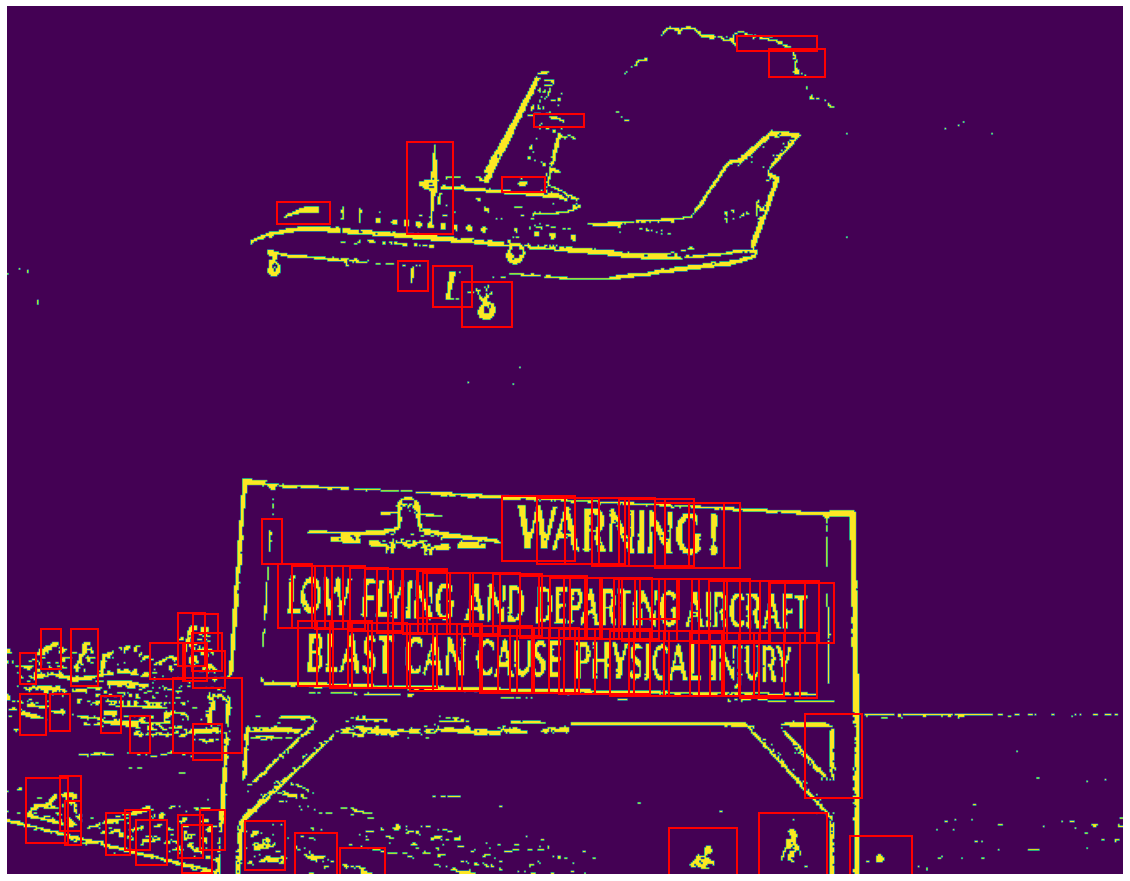### Step 4: Combine the overlapping boxes and remove the boxes which are not overlapping with any other box.

The idea I am using here is to combine boxes which are overlapping with each other and the ones which are almost in the same line with each other. I have listed down the code for each one of them below.

#### Check if two boxes are overlapping.

```def is_overlapping(box1, box2):
#     if (RectA.minc < RectB.maxc && RectA.maxc > RectB.minc &&
#      RectA.minr > RectB.maxr && RectA.maxr < RectB.minr )
if box1 < box2 and box1 > box2 and box1 < box2 and box1 > box2:
return True
return False
```

#### Check if the two boxes are in the same line.

```def is_almost_in_line(box1, box2):
centroid_b1 = [int((box1+box1)/2), int((box1+box1)/2)]
centroid_b2 = [int((box2+box2)/2), int((box2+box2)/2)]
if (centroid_b2-centroid_b1) == 0:
return True

angle = (np.arctan(np.abs((centroid_b2-centroid_b1)/(centroid_b2-centroid_b1)) )*180)/np.pi
if angle > 80:
return True

return False```

#### Combine two boxes which are overlapping each other.

```def combine_boxes(box1, box2):
minr = np.min([box1,box2])
minc = np.min([box1,box2])
maxr = np.max([box1,box2])
maxc = np.max([box1,box2])
return [minr, minc, maxr, maxc]```

#### Group the boxes into bigger boxes.

```def group_the_bounding_boxes(bounding_boxes):
stime = time.time()
number_of_checks = 0
box_groups = []
dont_check_anymore = []
for iindex, box1 in enumerate(bounding_boxes):
if iindex in dont_check_anymore:
continue

group_size = 0
bigger_box = box1

for jindex, box2 in enumerate(bounding_boxes):
if jindex in dont_check_anymore:
continue

if jindex == iindex:
continue

number_of_checks+=1

if is_overlapping(bigger_box, box2) and is_almost_in_line(bigger_box, box2):
bigger_box = combine_boxes(bigger_box, box2)
dont_check_anymore.append(jindex)
group_size += 1

if group_size > 0:
# check if this group overlaps any other
# and combine it there otherwise make a new box.
combined_with_existing_box = False
for kindex, box3 in enumerate(box_groups):
if is_overlapping(bigger_box, box3):
bigger_box = combine_boxes(bigger_box, box3)
box_groups[kindex] = bigger_box
combined_with_existing_box = True
break

if not combined_with_existing_box:
box_groups.append(bigger_box)

else:
dont_check_anymore.append(iindex)

print("number_of_checks:", number_of_checks)
print("time_taken:", str(time.time() - stime))
return box_groups```

Output from the above code is as shown below: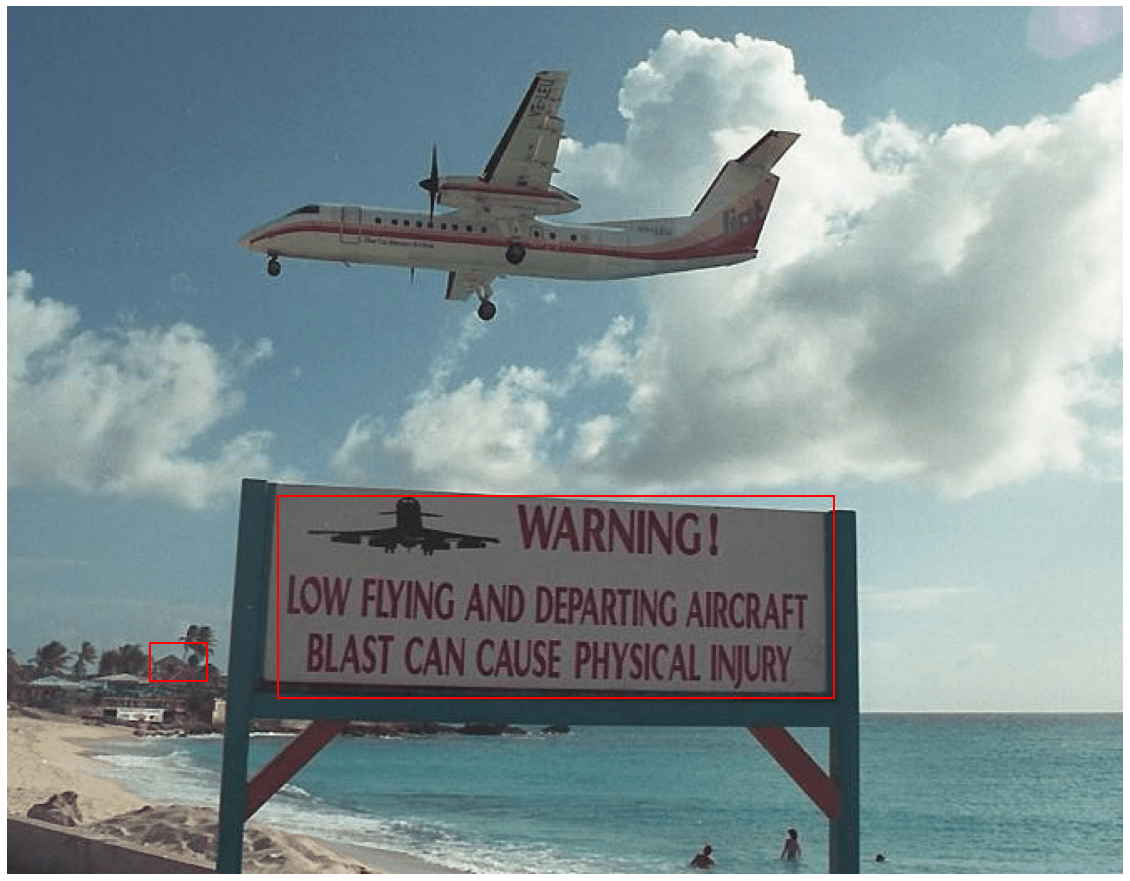Image extracted using the bounding box coordinates: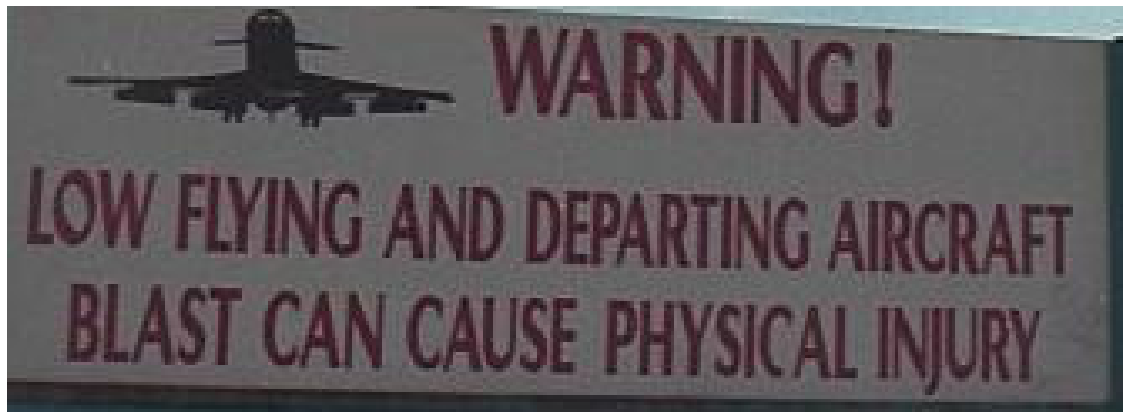As you can see in the image above, the text regions are now connected to one. You can pass this into an OCR system to extract text.

Other Samples:

Code for text detector : https://github.com/muthuspark/text-detector
Jupyter notebook: https://nbviewer.jupyter.org/github/muthuspark/text-detector/blob/master/notebooks/Text%20Segmentation%20in%20Image.ipynb

## References

B. Epshtein, E. Ofek and Y. Wexler, “Detecting text in natural scenes with stroke width transform,” 2010 IEEE Computer Society Conference on Computer Vision and Pattern Recognition, San Francisco, CA, 2010, pp. 2963-2970.

Tran, Tuan Anh Pham et al. “Separation of Text and Non-text in Document Layout Analysis using a Recursive Filter.” TIIS 9 (2015): 4072-4091.

Chen, Huizhong, et al. “Robust Text Detection in Natural Images with Edge-Enhanced Maximally Stable Extremal Regions.” Image Processing (ICIP), 2011 18th IEEE International Conference on. IEEE, 2011.